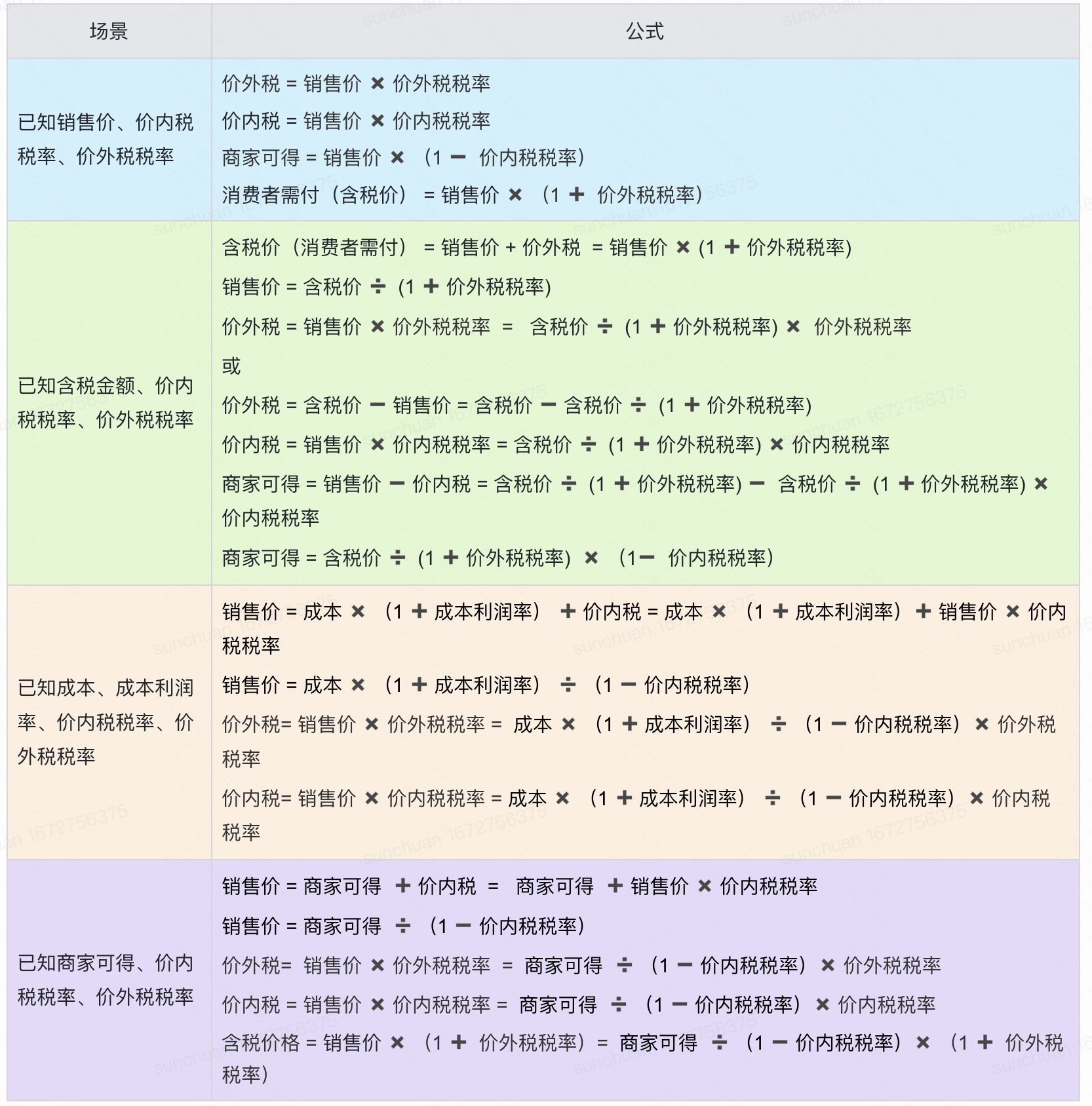n

nrsc

V1

2023/01/04阅读：25主题：橙心

# 价内税&价外税详细介绍

## 本文概要

• 本文将对价内税、价外税、含税价、不含税格等概念进行介绍
• 通过三个例题让你彻底明白计算价内税、价外税、商家可得金额、消费者应付金额的具体逻辑
• 对一些常用的税率公式进行了较为详细的推导和总结

## 价内税

`注意: 价内税由销售者承担 --- 销售者将其直接交到税务局`

``价内税 = 销售价 ✖️ 税率此时: 含税价 = 销售价不含税价 ＝ 含税价➖ 含税价 ✖️ 税率 = 含税价 ✖️ （1➖ 税率）含税价 = 不含税价 ➗ （1➖税率）``

## 价外税

`注意: 价外税由消费者承担 --- 销售者会替税务局收费，然后再交给税务局（消费者可以凭借发票去税务局抵扣）`

``价外税 = 销售价 ✖️ 税率不含税价 = 销售价 = 含税价 ➖ 价外税=》含税价 = 不含税价 ➕ 不含税价 ✖️ 税率 = 不含税价 ✖️ （1 ➕ 税率）=》不含税价 = 含税价 ➗ （1 + 税率）``

## 解题

### 例1

• 解题如下
``消费税（价内税） = 销售价格 ✖️ 税率 = 1000 ✖️ 20% = 200增值税（价外税） = 销售价格 ✖️ 税率 = 1000 ✖️ 13% = 130商家可得 = 销售价格 ➖ 价内税 = 1000 ➖ 200 = 800消费者需付价（含税价） = 销售价 ➕ 价外税 = 1000 ➕ 130 = 1130``
• 扩展一下

``商家可得 = 含税价➗（1 ➕ 价外税） ✖️ （1 ➖ 价内税）``
• 再扩展一下

``含税价 = 商家可得 ➗ （1 ➖ 价内税） ✖️ （1 ➕ 价外税）``

### 例2

• 解题如下
``含税价 = 销售价 + 价外税  = 销售价 ✖️ (1 ➕ 价外税税率)=> 销售价 = 含税价 ➗  (1 ➕ 价外税税率)=> 增值税（价外税）= 销售价 ✖️ 价外税税率  =   含税价 ➗  (1 ➕ 价外税税率) ✖️  价外税税率 = 5650  ➗  (1 ➕ 13%)  ✖️ 13% = 650或=> 增值税（价外税） = 含税价 ➖ 销售价 = 含税价 ➖ 含税价 ➗  (1 ➕ 价外税税率)  = 5650 ➖ 5650  ➗  (1 ➕ 13%)   =  650=> 消费税（价内税） = 销售价 ✖️ 价内税税率 = 含税价 ➗  (1 ➕ 价外税税率) ✖️ 价内税税率 = 5650  ➗  (1 ➕ 13%) ✖️ 10% = 500=> 商家可得 = 销售价 ➖ 价内税 = 含税价 ➗  (1 ➕ 价外税税率) ➖  含税价 ➗  (1 ➕ 价外税税率) ✖️ 价内税税率=> 商家可得 = 含税价 ➗  (1 ➕ 价外税税率)  ✖️ （1➖  价内税税率）= 5650 ➗  (1 ➕ 13%)  ✖️ （1 ➖ 10%）=  4500``

### 例3

• 前置知识
``成本 + 成本 ✖️ 成本利润率  = 商家可得``

• 解题如下
``销售价 = 成本 ✖️ （1 ➕ 成本利润率） ➕ 价内税 = 成本 ✖️ （1 ➕ 成本利润率）➕ 销售价 ✖️ 价内税税率=》销售价 = 成本 ✖️ （1 ➕ 成本利润率） ➗ （1 ➖ 价内税税率） =  5000 ✖️ （1 ➕ 15%） ➗ (1 ➖ 20% ) = 7187.5=》增值税（价外税）= 销售价 ✖️ 价外税税率 = 7187.5   ✖️  13% = 934.375=》消费税（价内税） = 销售价 ✖️ 价内税税率 =  7187.5   ✖️  20% = 1437.5=》商家可得 =  销售价  ✖️  (1 ➖ 价内税税率) =  7187.5  ✖️ （1 ➖ 20%） =  5750=》消费者需付 = 销售价 ✖️  (1 ➕ 价外税税率) =  7187.5  ✖️ （1 ➕ 13%）= 8121.875``

## 公式总结n
V1